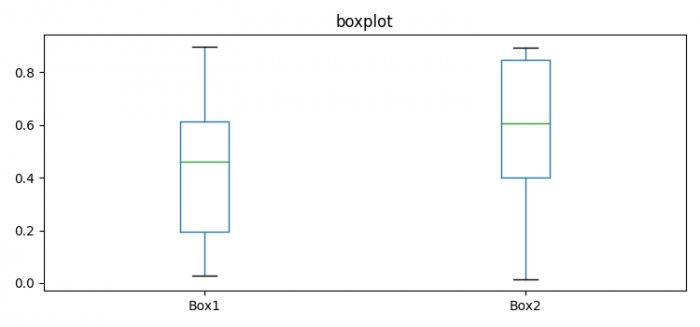# Plot multiple boxplots in one graph in Pandas or Matplotlib

To plot multiple boxplots in one graph in Pandas or Matplotlib, we can take the following steps −

## Steps

• Set the figure size and adjust the padding between and around the subplots.

• Make a Pandas data frame with two columns.

• Plot the data frame using plot() method, with kind='boxplot'.

• To display the figure, use show() method.

## Example

import pandas as pd
import numpy as np
from matplotlib import pyplot as plt

# Set the figure size
plt.rcParams["figure.figsize"] = [7.50, 3.50]
plt.rcParams["figure.autolayout"] = True

# Pandas dataframe
data = pd.DataFrame({"Box1": np.random.rand(10), "Box2": np.random.rand(10)})

# Plot the dataframe
ax = data[['Box1', 'Box2']].plot(kind='box', title='boxplot')

# Display the plot
plt.show()

## Output

It will produce the following output −Updated on: 13-Sep-2023

25K+ Views